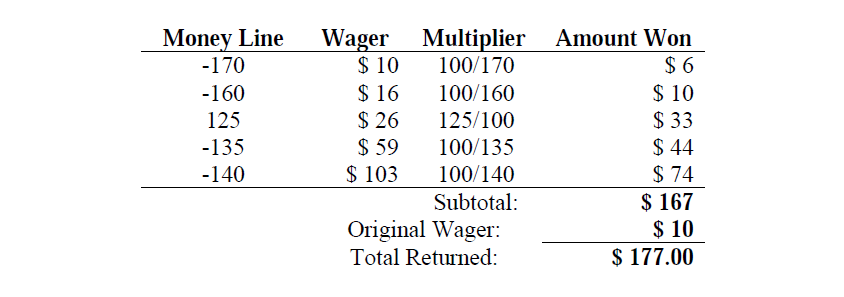# 2835. $10 to Win? 单点时限: 5.0 sec 内存限制: 256 MB Howdy Michael is a young and inexperienced gambler. Howdy bets much money in several Sport Games (basketball, baseball, hockey and football). This type of bet (combination of several Sport Games) is well-known like Parlay (gambling). Parlay (gambling) Rules A parlay wager is a combination of individual independent wagers that only pays if no individual wager loses. The payout from each wager is applied or “parlayed” to the next wager in turn. If any individual wager loses, the bettor receives nothing. If any individual wager is a tie or “push”, that wager is effectively ignored, reducing the ultimate payout. How to calculate payment of a Parlay (gambling)? The sports book quotes the payout rate for an individual wager as a “money line”, a nonzero integer in the range -2000 to 2000. To compute the payout for a successful wager, the money line is converted to a decimal multiplier as follows: if the money line is positive, it is divided by 100 to obtain the multiplier. If the money line is negative, 100 is divided into the absolute value to obtain the multiplier. The multiplier is always rounded to three digits after the decimal point. The wager is multiplied by this multiplier to determine the amount won. The amount won is always rounded to next value integer if the amounts won contains cents (no cents). Consider the following example for a five-way parlay wager:The maximum payout for any parlay wager is$1 million. If the calculated total exceeds that amount, the actual total returned will be $1 million. Write a program that helps us to Howdy Michael will calculate the total amount returned for a series of parlay wagers. For each parlay wager, your program is to print the total amount returned in dollars and cents on a single line starting in the first column without embedded or trailing whitespace. Print the leading dollar sign and insert commas at the millions and thousands positions as needed. ### 输入格式 Input will consist of several wagers. The first line of input to your program will contain the total number of parlay wagers as a single positive integer. Each wager that follows will be represented by a series of lines. The first line of each parlay wager contains the initial bet and the count of individual wagers as integers separated from each other by a single space. The following lines represent the individual wagers, one per line. Each individual wager is given as its money line. ### 输出格式 For each parlay wager, you should generate one line of output with the following values: The dataset number as a decimal integer (start counting at one), a space, and the total amount returned in dollars followed by “.00” cents. Print the leading dollar sign and insert commas at the millions and thousands positions as needed. ### 样例 Input 3 10 5 -170 -160 125 -135 -140 10 8 -170 -160 125 -135 -140 110 120 130 15 5 100 -100 -250 135 -350  Output 1$177.00
2 $1,884.00 3$255.00


23 人解决，42 人已尝试。

35 份提交通过，共有 247 份提交。

5.9 EMB 奖励。